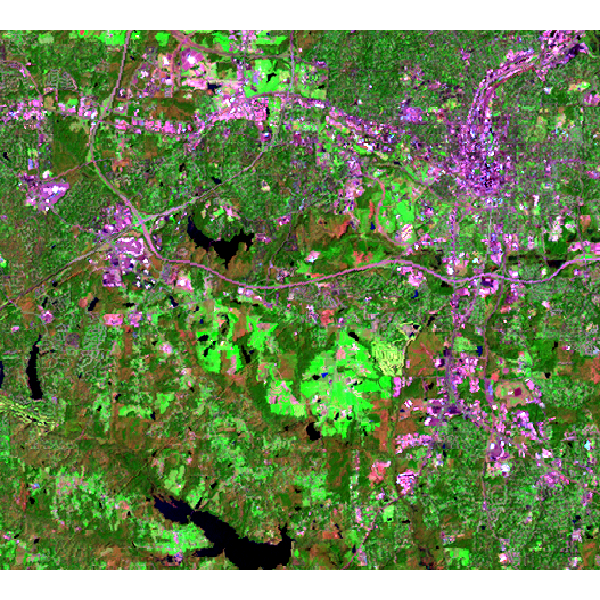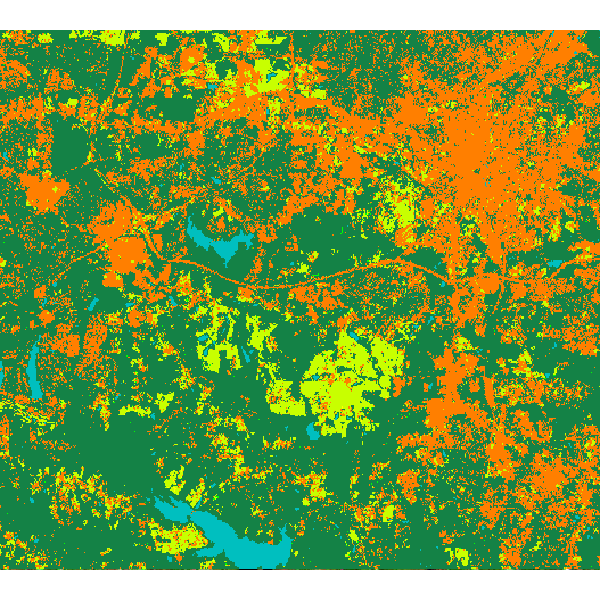## NAME

r.learn.train - Supervised classification and regression of GRASS rasters using the python scikit-learn package.

## KEYWORDS

raster, classification, regression, machine learning, scikit-learn, training, parallel

## SYNOPSIS

r.learn.train
r.learn.train --help
r.learn.train [-fsb] group=name [training_map=name] [training_points=name] [field=name] save_model=name [model_name=string] [penalty=string[,string,...]] [alpha=float[,float,...]] [l1_ratio=float[,float,...]] [c=float[,float,...]] [epsilon=float[,float,...]] [max_features=integer[,integer,...]] [max_depth=integer[,integer,...]] [min_samples_leaf=integer[,integer,...]] [n_estimators=integer[,integer,...]] [learning_rate=float[,float,...]] [subsample=float[,float,...]] [n_neighbors=integer[,integer,...]] [hidden_units=string[,string,...]] [weights=string[,string,...]] [group_raster=name] [cv=integer] [preds_file=name] [classif_file=name] [category_maps=name[,name,...]] [fimp_file=name] [param_file=name] [random_state=integer] [n_jobs=integer] [save_training=name] [load_training=name] [--overwrite] [--help] [--verbose] [--quiet] [--ui]

### Flags:

-f
Compute Feature importances
Compute feature importances using permutation
-s
Standardization preprocessing
Standardize feature variables (convert values the get zero mean and unit variance)
-b
Balance training data using class weights
Automatically adjust weights inversely proportional to class frequencies
--overwrite
Allow output files to overwrite existing files
--help
Print usage summary
--verbose
Verbose module output
--quiet
Quiet module output
--ui
Force launching GUI dialog

### Parameters:

group=name [required]
Group of raster layers to be classified
GRASS imagery group of raster maps representing predictor variables to be used in the machine learning model
training_map=name
Labelled pixels
Raster map with labelled pixels for training
training_points=name
Vector map with training samples
Vector points map where each point is used as training sample
field=name
Response attribute column
Name of attribute column in training_points table containing response values
save_model=name [required]
Save model to file (for compression use e.g. '.gz' extension)
Name of file to store model results using python joblib
model_name=string
model_name
Supervised learning model to use
Default: RandomForestClassifier
penalty=string[,string,...]
The regularization method
The regularization method to be used for the SGDClassifier and SGDRegressor
Options: l1, l2, elasticnet
Default: l2
alpha=float[,float,...]
Constant that multiplies the regularization term
Constant that multiplies the regularization term for SGDClassifier/SGDRegressor/MLPClassifier/MLPRegressor
Default: 0.0001
l1_ratio=float[,float,...]
The Elastic Net mixing parameter
The Elastic Net mixing parameter for SGDClassifier/SGDRegressor
Default: 0.15
c=float[,float,...]
Inverse of regularization strength
Inverse of regularization strength (LogisticRegression and SVC/SVR)
Default: 1.0
epsilon=float[,float,...]
Epsilon in the SVR model
Epsilon in the SVR model
Default: 0.1
max_features=integer[,integer,...]
Number of features available during node splitting; zero uses estimator defaults
Number of features available during node splitting (tree-based classifiers and regressors)
Default: 0
max_depth=integer[,integer,...]
Maximum tree depth; zero uses estimator defaults
Maximum tree depth for tree-based method; zero uses estimator defaults (full-growing for Decision trees and Randomforest, 3 for GBM)
Default: 0
min_samples_leaf=integer[,integer,...]
The minimum number of samples required to form a leaf node
The minimum number of samples required to form a leaf node in tree-based estimators
Default: 1
n_estimators=integer[,integer,...]
Number of estimators
Number of estimators (trees) in ensemble tree-based estimators
Default: 100
learning_rate=float[,float,...]
learning rate
learning rate (also known as shrinkage) for gradient boosting methods
Default: 0.1
subsample=float[,float,...]
The fraction of samples to be used for fitting
The fraction of samples to be used for fitting, controls stochastic behaviour of gradient boosting methods
Default: 1.0
n_neighbors=integer[,integer,...]
Number of neighbors to use
Number of neighbors to use
Default: 5
hidden_units=string[,string,...]
Number of neurons to use in the hidden layers
Number of neurons to use in each layer, i.e. (100;50) for two layers
Default: (100;100)
weights=string[,string,...]
weight function
Distance weight function for k-nearest neighbours model prediction
Options: uniform, distance
Default: uniform
group_raster=name
Custom group ids for training samples from GRASS raster
GRASS raster containing group ids for training samples. Samples with the same group id will not be split between training and test cross-validation folds
cv=integer
Number of cross-validation folds
Number of cross-validation folds
Default: 1
preds_file=name
Save cross-validation predictions to csv
Name of output file in which to save the cross-validation predictions
classif_file=name
Save classification report to csv
Name of output file to save the classification report
category_maps=name[,name,...]
Names of categorical rasters within the imagery group
Names of categorical rasters within the imagery group that will be one-hot encoded. Leave empty if none.
fimp_file=name
Save feature importances to csv
Name for output file
param_file=name
Save hyperparameter search scores to csv
Name of file to save the hyperparameter tuning results
random_state=integer
Seed to use for random state
Seed to use for random state to enable reproducible results for estimators that have stochastic components
Default: 1
n_jobs=integer
Number of cores for multiprocessing
Number of cores for multiprocessing, -2 is n_cores-1
Default: -2
save_training=name
Save training data to csv
Name of output file to save training data in comma-delimited format
Load previously extracted training data from a csv file

## DESCRIPTION

r.learn.train performs training data extraction, supervised machine learning and cross-validation using the python package scikit learn. The choice of machine learning algorithm is set using the model_name parameter. For more details relating to the classifiers, refer to the scikit learn documentation. The training data can be provided either by a GRASS raster map containing labelled pixels using the training_map parameter, or a GRASS vector dataset containing point geometries using the training_points parameter. If a vector map is used then the field parameter also needs to indicate which column in the vector attribute table contains the labels/values for training.

For regression models the field parameter must contain only numeric values. For classification models the field can contain integer-encoded labels, or it can represent text categories that will automatically be encoded as integer values (in alphabetical order). These text labels will also be applied as categories to the classification output when using r.learn.predict. The vector map should also not contain multiple geometries per attribute.

### Supervised Learning Algorithms

The following classification and regression methods are available:

Model Description
LogisticRegression, LinearRegression Linear models for classification and regression
SGDClassifier, SGDRegressor Linear models for classification and regression using stochastic gradient descent optimization suitable for large datasets. Supports l1, l2 and elastic net regularization
KNeighborsClassifier, KNeighborsRegressor Local approximation methods for classification/regression that assign predictions to new observations based on the values assigned to the k-nearest observations in the training data feature space
GaussianNB Gaussian Naive Bayes algorithm and can be used for classification
DecisionTreeClassifier DecisionTreeRegressor Classification and regression tree models that map observations to a response variable using a hierarchy of splits and branches. The terminus of these branches, termed leaves, represent the prediction of the response variable. Decision trees are non-parametric and can model non-linear relationships between a response and predictor variables, and are insensitive the scaling of the predictors
RandomForestClassifier, RandomForestRegressor, ExtraTreesClassifier, ExtraTreesRegressor Ensemble classification and regression tree methods. Each tree in the ensemble is based on a random subsample of the training data. Also, only a randomly-selected subset of the predictors are available during each node split. Each tree produces a prediction and the final result is obtained by averaging across all of the trees. The ExtraTreesClassifier and ExtraTreesRegressor are variant on random forests where during each node split, the splitting rule that is selected is based on the best of a several randomly-generated thresholds
SVC, SVR Support Vector Machine classifiers and regressors. Only a linear kernel is enabled in r.learn.ml2 because non-linear kernels are too slow for most remote sensing and spatial datasets
MLPClassifier, MLPRegressor Multi-layer perceptron algorithm for classification or regression

### Hyperparameters

The estimator settings tab provides access to the most pertinent parameters that affect the previously described algorithms. The scikit-learn estimator defaults are generally supplied, and these parameters can be tuned using a grid-search by inputting multiple comma-separated parameters. The grid search is performed using a 3-fold cross validation. This tuning can also be accomplished simultaneously with nested cross-validation by settings the cv option to > 1.

The following table summarizes the hyperparameter and which models they apply to:

Hyperparameter Description Method
alpha The constrant used to multiply the regularization term SGDClassifier, SGDRegressor, MLPClassifier, MLPRegressor
l1_ratio The elastic net mixing ration between l1 and l2 regularization SGDClassifier, SGDRegressor
c Inverse of the regularization strength LogisticRegression, SVC, SVR
epsilon Width of the margin used to maximize the number of fitted observations SVR
max_features The number of predictor variables that are randomly selected to be available at each node split RandomForestClassifier, RandomForestRegressor, ExtraTreesClassifier, ExtraTreesRegressor, GradientBoostingClassifier, GradientBoostingRegressor, HistGradientBoostingClassifier, HistGradientBoostingRegressor
hidden_units The number of neurons in each hidden layer, e.g. (100;100) for 100 neurons in two hidden layers. Tuning can be performed using comma-separated values, e.g. (100;100),(200;200). MLPClassifier, MLRRegressor

### Preprocessing

Although tree-based classifiers are insensitive to the scaling of the input data, other classifiers such as linear models may not perform optimally if some predictors have variances that are orders of magnitude larger than others. The -s flag adds a standardization preprocessing step to the classification and prediction to reduce this effect. Additionally, most of the classifiers do not perform well if there is a large class imbalance in the training data. Using the -b flag balances the training data by weighting of the minority classes relative to the majority class. This does not apply to the Naive Bayes or LinearDiscriminantAnalysis classifiers.

Scikit learn does not specifically recognize raster predictors that represent non-ordinal, categorical values, for example if using a landcover map as a predictor. Predictive performances may be improved if the categories in these maps are one-hot encoded before training. The parameter categorical_maps can be used to select rasters that in contained within the imagery group to apply one-hot encoding before training.

### Feature Importances

In addition to model fitting and prediction, feature importances can be generated using the -f flag. The feature importances method uses a permutation-based method can be applied to all the estimators. The feature importances represent the average decrease in performance of each variable when permuted. For binary classifications, the AUC is used as the metric. Multiclass classifications use accuracy, and regressions use R2.

### Cross-Validation

Cross validation can be performed by setting the cv parameters to > 1. Cross-validation is performed using stratified k-folds for classification and k-folds for regression. Several global and per-class accuracy measures are produced depending on whether the response variable is binary or multiclass, or the classifier is for regression or classification. Cross-validation can also be performed in groups by supplying a raster containing the group_ids of the partitions using the group_raster option. In this case, training samples with the same group id as set by the group_raster will never be split between training and test partitions during cross-validation. This can reduce problems with overly optimistic cross-validation scores if the training data are strongly spatially correlated, i.e. the training data represent rasterized polygons.

## NOTES

Many of the estimators involve a random process which can causes a small amount of variation in the classification/regression results and and feature importances. To enable reproducible results, a seed is supplied to the estimator. This can be changed using the randst parameter.

For convenience when repeatedly training models on the same data, the training data can be saved to a csv file using the save_training option. This data can then imported into subsequent classification runs, saving time by avoiding the need to repeatedly query the predictors.

## EXAMPLE

Here we are going to use the GRASS GIS sample North Carolina data set as a basis to perform a landsat classification. We are going to classify a Landsat 7 scene from 2000, using training information from an older (1996) land cover dataset.

Landsat 7 (2000) bands 7,4,2 color composite example:Note that this example must be run in the "landsat" mapset of the North Carolina sample data set location.

First, we are going to generate some training pixels from an older (1996) land cover classification:

```g.region raster=landclass96 -p
r.random input=landclass96 npoints=1000 raster=training_pixels
```

Then we can use these training pixels to perform a classification on the more recently obtained landsat 7 image:

```# train a random forest classification model using r.learn.train
r.learn.train group=lsat7_2000 training_map=training_pixels \
model_name=RandomForestClassifier n_estimators=500 save_model=rf_model.gz

# perform prediction using r.learn.predict

# check raster categories - they are automatically applied to the classification output
r.category rf_classification

# copy color scheme from landclass training map to result
r.colors rf_classification raster=training_pixels
```

Random forest classification result:r.learn.ml2 (overview), r.learn.predict

## REFERENCES

Scikit-learn: Machine Learning in Python, Pedregosa et al., JMLR 12, pp. 2825-2830, 2011.

Steven Pawley

## SOURCE CODE

Available at: r.learn.train source code (history)

Latest change: Wednesday Sep 20 13:55:24 2023 in commit: fb8fbe7f92061801e51d853f69ffc3e3438b0718

© 2003-2023 GRASS Development Team, GRASS GIS 8.3.1dev Reference Manual